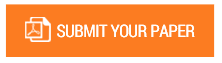Modeling and Performance Analysis of Perturb and Observe (P&O), Incremental Conductance (IC), Fuzzy Logic, and Model Predictive MPPT Controllers Using a Boost Converter

Authors : K.Hareesh Reddy; R.Vijayasanthi; K.RamaSudha

Volume/Issue : Volume 5 - 2020, Issue 12 - December

Scribd : https://bit.ly/37UK6zN

In a photovoltaic module, there is a single operating point at which maximum output can be drawn from the module. This point at which maximum power can be obtained is known as “Maximum Power Point (MPP)”. There is need to find the MPP and ensure that the point of operation is close to and around this point. The process of finding the MPP and always trying to keep the PV panel operating point at MPP is called “Maximum Power Point Tracking (MPPT)”.The objective of the current paper is to model the PV cell and present the modeling of four different control techniques using MATLAB. The four control techniques are Perturb and Observe (P&O), Incremental Conductance (IC), Fuzzy Logic Control (FLC), and Model Predictive Control (MPC) algorithms. These algorithms are used to track the MPP of the PV module. All the control algorithms function together with a DC-DC converter, in this paper, a BOOST converter is used to perform all the simulations and maximum power point tracking. The P-V and I-V characteristics are drawn with the cell temperature kept constant. This paper also presents the study of the four control techniques and their performance analysis. The outcomes of these four techniques are compared, and relevant conclusions are drawn.

#### CALL FOR PAPERS

Paper Submission Last Date
31 - May - 2022